# How To Force Text String to Upper,Lower, or Proper Case in Excel

This post will guide you how to force test strings to uppercase or lowercase or Proper cases through an Excel formula or data validation in your Excel 2013/2016. How to convert text to upper, lower, or proper case in Excel.

Assuming that you want to force text strings to Upper case when you type words into one cell, and how to do it. You can use an Excel function in Data Validation feature to achieve it. Just do the following steps:

Step1: go to DATA tab, click Data Validation command under the Data Tools group. Then clicking on the Data Validation menu from the drop down menu list. Then the Data Validation window will appear.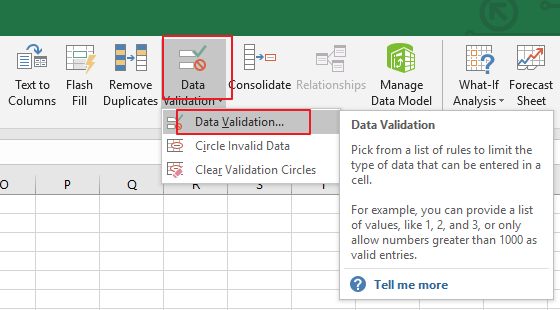Step2: in the Data Validation dialog box, and switch to Settings tab, and click the Allow drop down list and choose Custom value from the drop down menu list.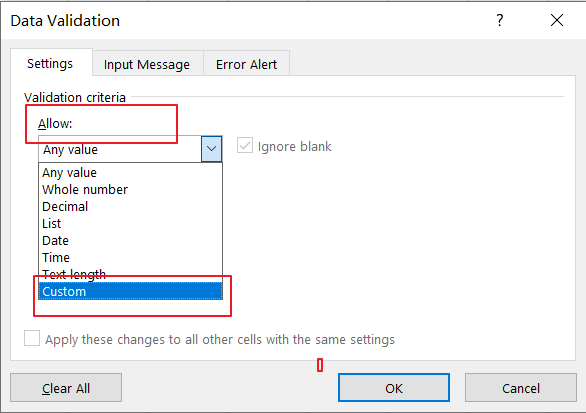Step3: Then type the following formula in the Formula text box in the Data Validation dialog box.

Note:

If you want to convert text string to Upper Case, type the following formula:

=EXACT(UPPER(B1),B1)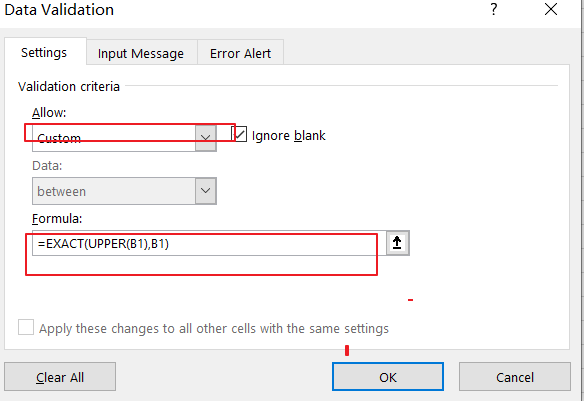If you want to convert text string to Lower Case, just use the following formula:

=EXACT(LOWER(B1),B1)

If you want to convert text string to Proper Case, just use the following formula:

=EXACT(PROPER(B1),B1)

Step4: then you need to switch to Error Alert tab in the Data Validation dialog box. And select Stop value from the Style drop down list box, and type some error message under Error message text box. Then click on Ok button.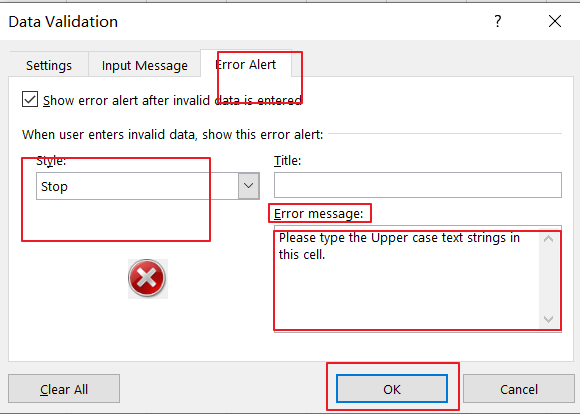Step5: you can try to enter one text string in cell B1 to verify if it take effect.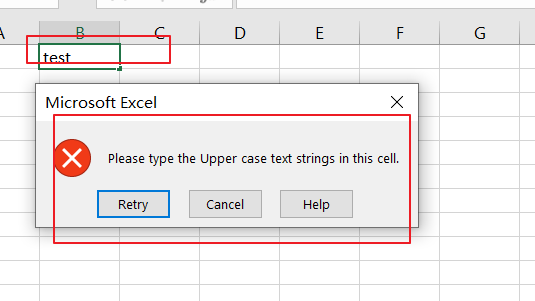You can also use an Excel VBA Macro to achieve the same result of forcing Text string to Upper, Lower or Proper cases in you worksheet. Just do the following steps:

Step1: open your excel workbook and then click on “`Visual Basic`” command under `DEVELOPER` Tab, or just press “`ALT+F11`” shortcut.Step2: then the “`Visual Basic Editor`” window will appear.

Step3: choose on your current worksheet from the left Project Explorer, and double click on it to open the code window, and the copy and paste the following VBA code into it.

For Upper Case:

```Private Sub Worksheet_Change(ByVal Target As Range)

Target.Value = VBA.UCase(Target.Value)

End Sub```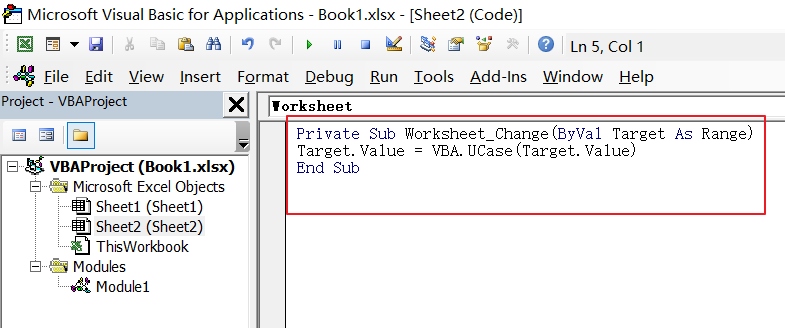For Lower Case:

```Private Sub Worksheet_Change(ByVal Target As Range)

Target.Value = VBA. LCase (Target.Value)

End Sub```

For Proper Case:

```Private Sub Worksheet_Change(ByVal Target As Range)

Target.Value = Application.WorksheetFunction.Proper(Target.Value)

End Sub```

Step4: save and close the current VBA window. And then you can try to enter one text string to verify it. when you type one word when it is lower or proper cases, and it will convert it to Uppercase.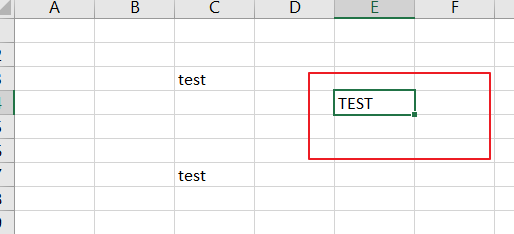### Related Functions

• Excel UPPER function
The Excel UPPER function converts all characters in text string to uppercase.The UPPER function is a build-in function in Microsoft Excel and it is categorized as a Text Function.The syntax of the UPPER function is as below:= UPPER (text)…
• Excel LOWER function
The Excel LOWER function converts all characters in a text string to lowercase.The syntax of the LOWER function is as below:= LOWER  (text)…
• Excel Proper Function
The Excel PROPER function capitalizes the first character in each word of a text string and set other characters to lowercase. The syntax of the PROPER function is as below:= PROPER  (text)…
• Excel EXACT function
The Excel EXACT function compares if two text strings are the same and returns TRUE if they are the same, Or, it will return FALSE.The syntax of the EXACT function is as below:= EXACT (text1,text2)…

Related Posts

Find and Replace Multiple Values

This post will guide you how to find and replace multiple values at once with VBA macro or using formula  in Excel. How do I make multiple find and replace in Excel. Suppose that you have a few cells containing ...

VBA Macro For VLOOKUP From Another Sheet

In the previous post, you should know that how to fix or remove the #N/A error when using VLOOKUP formula to lookup value from another sheet. And this post will show you how to use VBA code to vlookup data ...

How To Insert Comments in Protected Worksheet in Excel

This post will show you how to allow comments in a protected worksheet in Excel. You can easily to insert comments into cells in a normal worksheet in Excel, but if want to insert a comment in a worksheet that ...

How To Convert Text to Upper Cases(Using VBA) in Excel

This post will show you how to switch from lower case to upper case in Excel. and I am going to show you two different ways of converting text to upper cases using formula or VBA macro in Excel 2013,Excel ...

How To Hide Every Other Row in Excel (Using VBA)

This post will show you how to hide alternate rows or columns in Excel or how to hide every third, fourth, fifth row or column in Excel. If you want to hide every other row in your current worksheet, how ...

How to Disable the Save As Prompt in Excel

This post will show you how to use a VBA Macro to save an Excel file and overwrite any existing file without a prompt so that you are going to get the little window that says file already exists do ...

How to Count Cells that Contain even or odd numbers in Excel

This post will guide you how to count the number of cells that contain odd or even numbers within a range of cells using a formula in Excel 2013/2016.How do I count cells that contain odd numbers through the use ...

How to Count Cells that Contain negative Numbers in Excel

This post will guide you how to count the number of cells that contain negative numbers within a range of cells using a formula in Excel 2013/2016.You can count the number of negative numbers in your data using easy functions ...

How to Count Cells Are Not Blank or Empty in Excel

This post will guide you how to count cells that are not blank or empty in a given range cells using a formula in Excel 2013/2016.How do I count the number of cells that are not blank in a particular ...

How to Count Cells Less Than a Specific Value in Excel

This post will guide you how to count the number of cells less than a particular numeric value in a given range cells using a formula in Excel 2013/2016. How do I count cells that are less than a specific ...

Sidebar